# Multiplying Decimals

Times up!
Times up! You may finish answering the current question and then proceed to your score!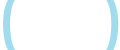Sorry, you have used all of your available hints for this lesson.
Practice Limit Reached

You've reached your daily practice limit of 12 questions.

Award InformationAwarded toQuestion Hint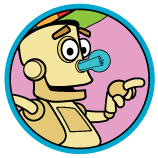Choose Family Member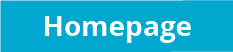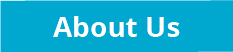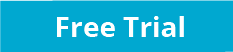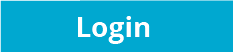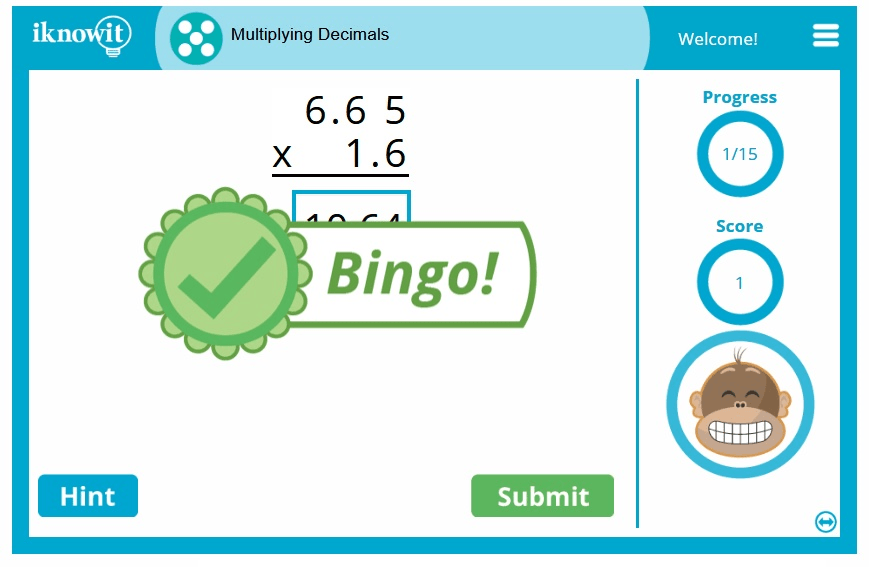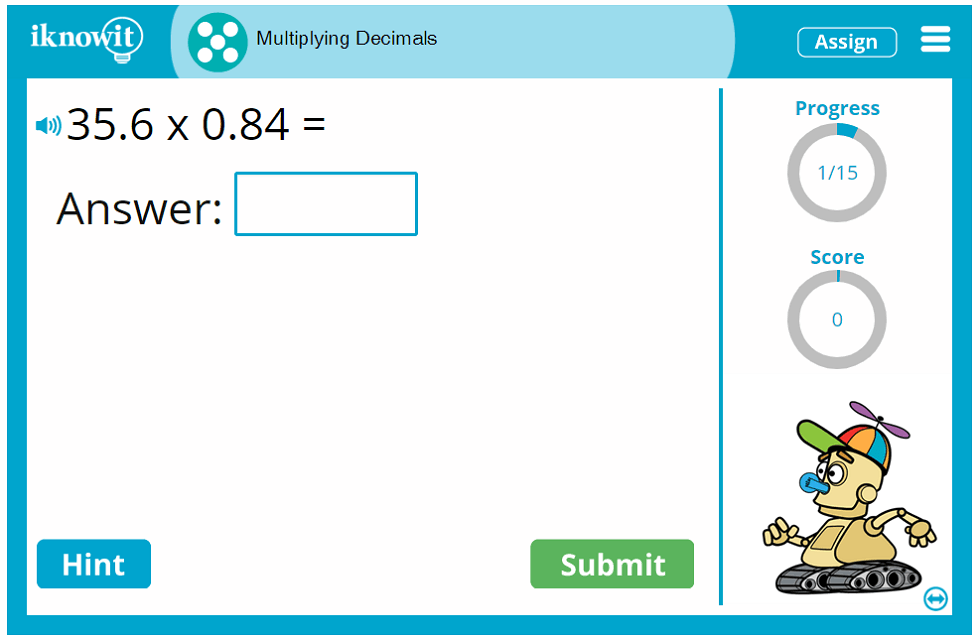## Math Lesson: Multiplying Decimals

This interactive math activity helps students practice multiplying decimals. In this math lesson, students will solve multiplication problems in which both of the products are numbers with decimals. The interactive multiplication problems are presented in a variety of question formats. Some questions are horizontal multiplication problems. Others are vertical multiplication problems. There are also a number of word problems in this multiplying decimals activity.

Students are allowed a specific number of hints in this multiplication lesson. The hint will help students figure out the first step in the multiplication problem. For example, the hint may say, "Step 1: multiply the tenths." The hint will also display a graphic with this first step of the multiplication question completed. When students have used up all their hints in a lesson, the hint window will display a message saying there are no more hints available.

One of the amazing features of this interactive multiplying decimals lesson is the detailed explanation page that appears when a student answers a question incorrectly. The explanation graphic contains a detailed breakdown of the multiplication problem, in four easy-to-follow steps. Multiply the tenths. Multiply the ones. Multiply the tens. Add the partial products. The explanation also includes important reminders, such as "Remember to regroup when necessary," and "Move the decimal three places." The thorough explanations in this engaging math lesson help students learn from their mistakes and improve their understanding of multiplication math skills.

When students are immersed this multiplying decimals math activity, they will feel like they are playing a fun, challenging math game. Teachers will see each "I Know It" lesson as an invaluable opportunity for their students to practice basic math skills. It's a win-win for students and teachers alike! You'll love the kid-friendly interface in this interactive math lesson. The questions are displayed in an easy-to-read format. The hint button is just a click away. In the upper-right corner of the screen, a progress icon tells students how many questions they've answered in the lesson. Below the progress icon, a score-keeper lets students know how many points they've accumulated so far as they solve the decimal multiplication questions correctly.

Another incredible feature of this multiplying decimals activity for kids is the audio option available for every question in the math lesson. Simply click the audio icon next to the question text, and the question will be read aloud in a clear voice. This option is great for students who have difficulty reading or students with ESL/ELL needs.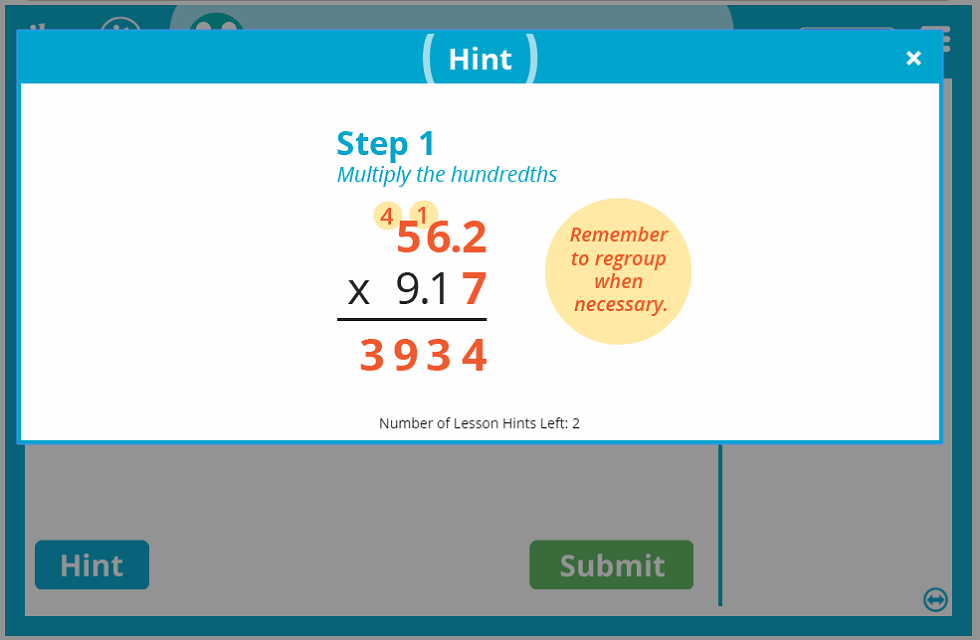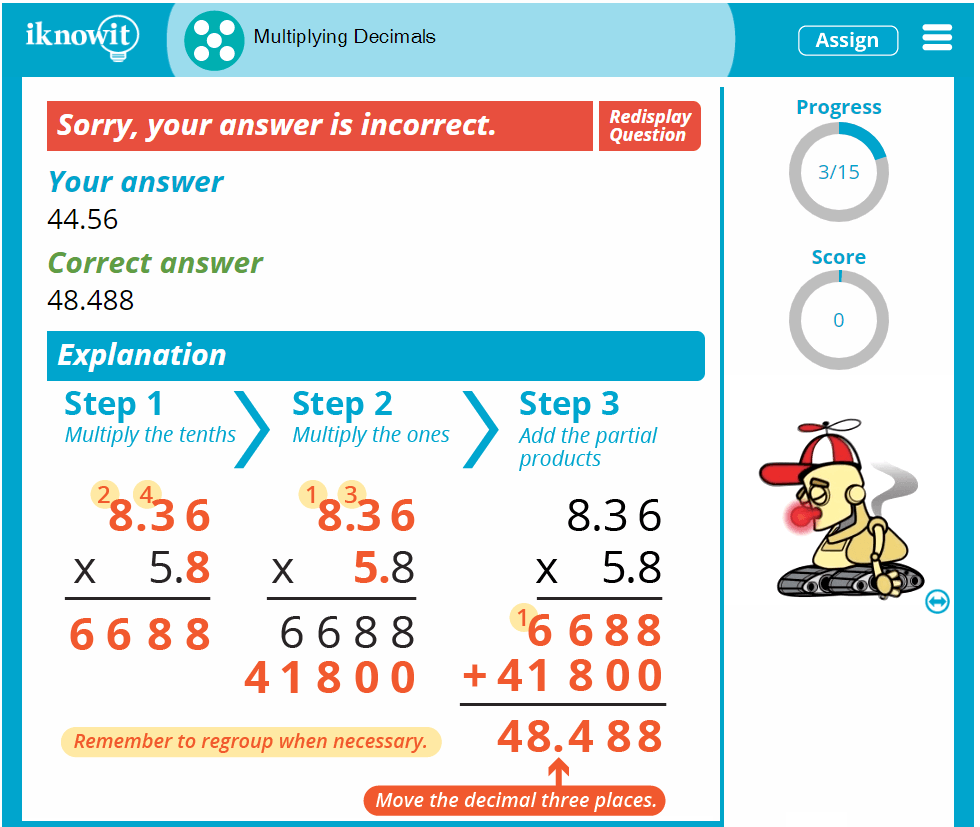## Free Lesson Preview versus "I Know It" Membership

Did you know you can try any "I Know It" lesson for free? That's right! The number of questions available for you to answer will be limited until you become a member of the website, but we're convinced you'll love the quality content in each of our math lessons, including multiplying decimals.

Besides the excellent variety and quality in the math activities themselves, we think you're also going to enjoy the administrative tools for teachers on "I Know It." Teachers can create student logins, view their students' scores for completed lessons, adjust lesson settings, and access student progress reports.

Additionally, educators are able to assign lessons based on the skill level and individual needs of each student. This is possible because grade levels on "I Know It" are alternately referred to as "Level A," "Level B," "Level C," instead of "1st Grade," "2nd Grade," "3rd Grade," etc. Students won't see a grade level attached to the lesson they're working on, making it easy for teachers to adjust settings to meet the child's educational needs.

We're excited for you and your students to try out this interactive multiplication game in your multiplying with decimals lesson. Will you give it a try today? Learning how to multiply with decimals has never been more fun than with math practice from "I Know It"! Remember to browse our growing collection of 5th grade math activities today!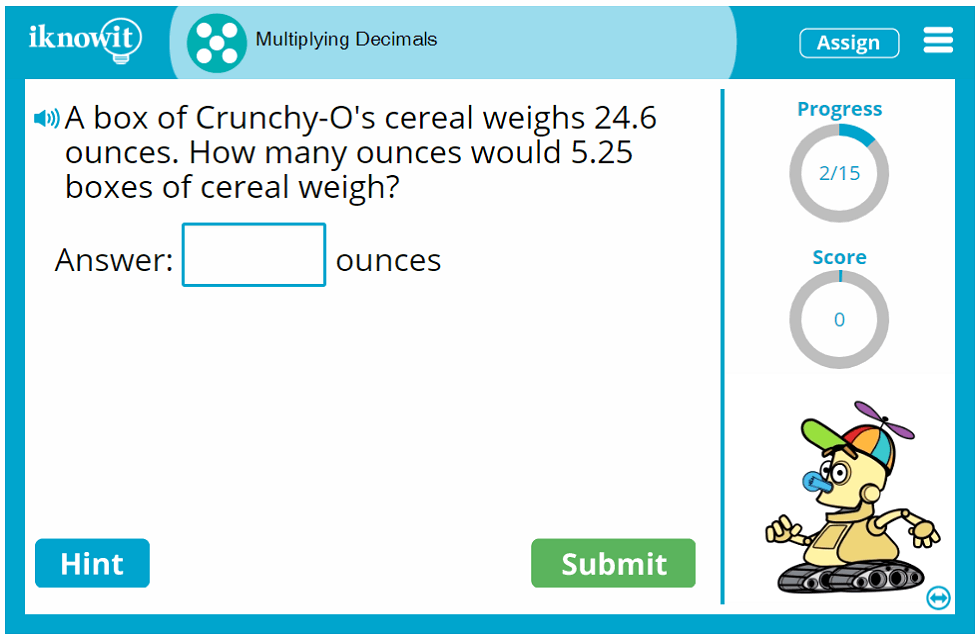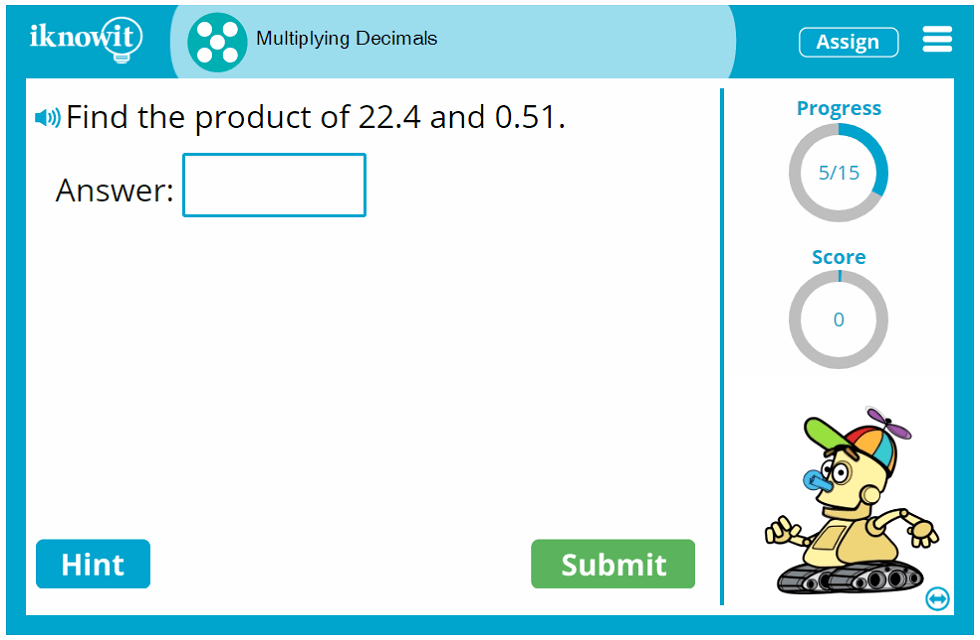## Level

We've categorized this multiplying decimals activity as a Level E lesson. It may be appropriate to use at a fourth grade or fifth grade level.

## Common Core Alignment

5.NBT.B.7
Number and Operations in Base Ten
Perform Operations with Multi-Digit Whole Numbers and with Decimals to Hundredths

Students should be able to add, subtract, multiply, and divide decimals to the hundredths place, using the assistance of concrete models or drawings to develop strategies based on place value and the properties of operations. Students should then be able to relate the strategy to a written method and explain the reasoning

## You might also be interested in...

Multiplying Decimals by Whole Numbers (Level E)
In this math activity, students will multiply decimal numbers by whole numbers. Regrouping and moving the decimal are math skills included in this lesson.

Multiplying 4-Digit by 2-Digit Numbers (Level D)
In this math game, students will multiply 4-digit numbers by 2-digit numbers. Questions are presented in a vertical, horizontal, and word problem format.